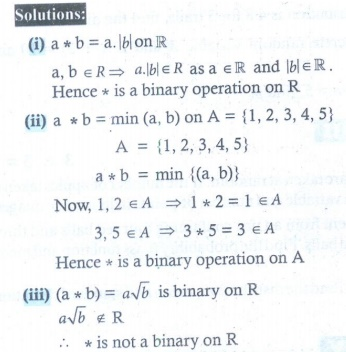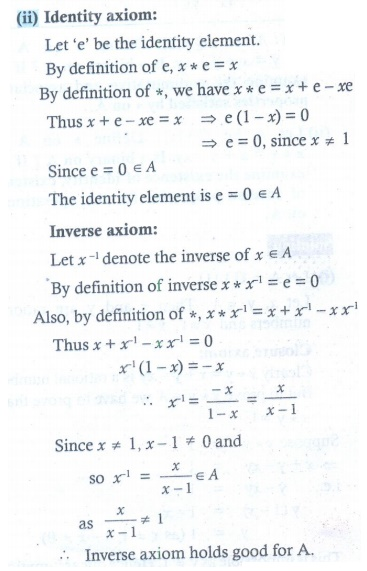Home | | Maths 12th Std | Exercise 12.1: Binary operations

# Exercise 12.1: Binary operations

Maths Book back answers and solution for Exercise questions - Mathematics : Discrete Mathematics: Binary operations: Exercise Problem Questions with Answer, Solution

EXERCISE 12.1

1. Determine whether  is a binary operation on the sets given below.

(i)  b = a. |b| on  (ii) a  b = min ( a , bon A = {1, 2, 3, 4, 5}

(iii) ( a  b) = a√b is binary on .2. On , define  by ( m  n)= mn + nm :  mn  . Is  binary on ?3. Let  be defined on  by ( a  b) = a + b + ab  7 . Is  binary on ℝ ? If so, find 3∗ (-7/15).4. Let A = {a + √5b : ab   } . Check whether the usual multiplication is a binary operation on .5. (i) Define an operation  on  as follows: a  b = ( a+b / 2); a , b  . Examine the closure, commutative, and associative properties satisfied by  on .

(ii) Define an operation  on  as follows: a  b = = ( a+b / 2); a , b  . Examine the existence of identity and the existence of inverse for the operation  on .6. Fill in the following table so that the binary operation  on A = {a , b , c} is commutative.7. Consider the binary operation  defined on the set A = {a , b, c, d} by the following table:Is it commutative and associative?8. Letbe any three boolean matrices of the same type. Find (i) A  B (ii) A  B (iii) ( A  B) C (iv) ( A  B) C .9. (i) Letand let  be the matrix multiplication. Determine whether M is closed under  . If so, examine the commutative and associative properties satisfied by  on M .

(ii) Letand let ∗ be the matrix multiplication. Determine whether M is closed under  . If so, examine the existence of identity, existence of inverse properties for the operation  on M.10. (i) Let A be \{1}. Define  on A by x  y = x + y − xy . Is  binary on A ? If so, examine the commutative and associative properties satisfied by  on A .

(ii) Let A be  \{1}. Define  on A by x  y = x + y − xy . Is  binary on A ? If so, examine the existence of identity, existence of inverse properties for the operation ∗ on A .(i) Yes, * is binary on  (ii) Yes, * is binary on A (iii) No, * is not binary on

2. No, * is not binary on

3. -88/15

4. Yes, usual multiplication is binary on A

5. (i) The given operation * is closure and commutative but not associative on .

(ii) Identity does not exist and so inverse does not exist.

6.7. No. The given operation is not commutative and associative

8.10. (i) It is commutative and associative. (ii) Identity and Inverse is exist.

Tags : Problem Questions with Answer, Solution , 12th Maths : UNIT 12 : Discrete Mathematics
Study Material, Lecturing Notes, Assignment, Reference, Wiki description explanation, brief detail
12th Maths : UNIT 12 : Discrete Mathematics : Exercise 12.1: Binary operations | Problem Questions with Answer, Solution